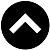-->

## MATHEMATICS FORM 2 SYLLABUS

1. Exponents
1. List the laws of exponents
2. Verify the laws of exponents
3. Apply laws of exponents in computations
2. Perform basic operations on radicals
3. Rationalize the denominator
4. Read square roots and cube roots of numbers from mathematical tables
3. Transposition of Formula
1. Re-arrange letters so that one letter is the subject of the formula
2. Transpose a formulae with square roots and square
2. Algebra
1. Binary Operations
1. Describe the binary operations
2. Perform binary operations
2. Brackets in Computation
1. Perform basic operations involving brackets
2. Simplify algebraic expressions involving the basic operations and brackets
1. Form a quadratic expression from two linear factors
2. Write the general form of quadratic expression
4. Factorization
1. Factorize linear expressions
1. Solving Equations
1. Determine the solution of a quadratic equation by factorization
2. Find the solution of a quadratic equation by completing the square
2. General Solution of Quadratic Equations
4. Logarithms
1. Standard Form
1. Write numbers in standard form
2. Perform computations which involved multiplication and division of numbers expressed in standard form
2. Laws Of Logarithms
1. State the laws of logarithms
2. Verify the laws of logarithms using the knowledge of exponents
3. Use the laws of Logarithms to simplify logarithmic expressions
4. Solve logarithmic equation
5. Apply laws of logarithms to find products, quotients, roots and powers of numbers
6. Apply logarithmic tables to find products and quotients of numbers computation
7. Apply logarithmic tables to find roots and power of numbers
5. Congruence
1. Congruence of Triangles
1. Determine the conditions for congruence of triangles
2. Prove congruence of triangle
3. Apply theorems on congruence of triangles to solve related problems
6. Similarity
1. Similar Figures
1. Identify similar polygons
2. Prove similarity theorems of triangles
7. Geometric And Transformations
1. Reflection
1. Describe the characteristics of reflection in a plane
2. Represent different reflections by drawings
2. Rotations
1. Describe characteristics of a rotation on a plane
2. Represent different rotation on a plane by drawings
3. Translation
1. State properties of translations
2. Represent translations drawings
4. Enlargement
1. Develop a scale of enlargement
2. Construct enlargement of a given figures
3. Draw figures to scale
4. Find actual distances represented by a scale drawings
5. Combined Transformations
1. Draw combined transformations
2. Solve simple problems on combined transformations
8. Pythagoras Theorem
1. Proof of Pythagoras Theorem
1. Prove the Pythagoras theorem
2. Application of Pythagoras Theorem
1. Apply the Pythagoras theorem to solve daily life problems
9. Trigonometry
1. Trigonometric Rations
1. Define sine, cosine and tangent of an angle using a right angled triangle
2. Trigonometric Ratios of Special Angles
1. Determine the sine, cosine and tangent of 30°, 45° and 60° without using mathematical tables
2. Solve simple trigonometric problems related to special angles
3. Trigonometric Tables
1. Read the trigonometric ratios from tables
2. Solve problems involving trigonometric ratios from tables
4. Angles of Elevation and Depression
1. Demonstrate angles of elevation and angles of depression
2. Solve Problems involving angles of elevation and angles of depression
10. Sets
1. Description of a Set
1. Define a set
2. List the members of a set
3. Name a set
4. Distinguish sets by listing and by stating the members
2. Types of Sets
1. Define a universal set and an empty set
2. Distinguish between finite and infinite sets
3. Distinguish between equivalent and equal sets
3. Subsets
1. Define a subset
2. List subsets of a given set
3. Distinguish between proper and improper subsets
4. Calculate the number of subsets in a set
4. Operations With Sets
1. Find union of two sets
2. Find the compliment of a set
3. Find the number of elements in the union and intersection of two sets
5. Venn Diagrams
1. Represent a sets by using venn diagrams
2. Interpret information from venn diagrams
11. Statistics
1. Pictograms
1. Display Information by pictograms
2. Interpret pictograms
2. Bar Charts
1. Draw horizontal and vertical bar charts
2. Interpret bar chart
3. Line Graphs
1. Represent data using line graphs
2. Interpret line graphs
4. Pie Chart
1. Display data using pie charts
2. Interpret pie charts
5. Frequency Distribution Tables
1. Make frequency distribution tables from raw data
2. Interpret frequency distribution table form raw data
3. Interpret frequency distribution tables
6. Frequency Polygons
1. Draw frequency polygons from frequency distribution tables
2. Interpret frequency polygons
7. Histograms
1. Draw histograms from frequency distribution table
2. Interpret histograms
8. Cumulative Frequency Curves
1. Draw cumulative frequency curves from a cumulative frequency distribution table
2. Interpret a cumulative frequency curve